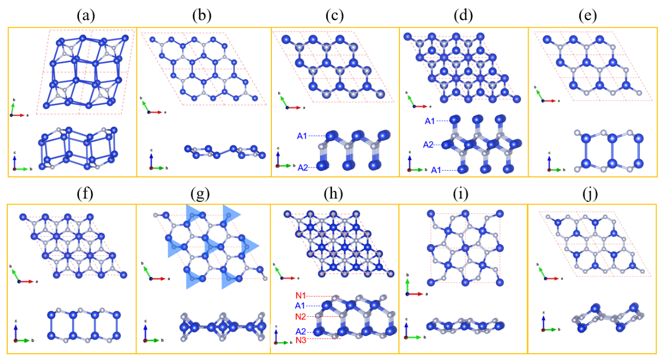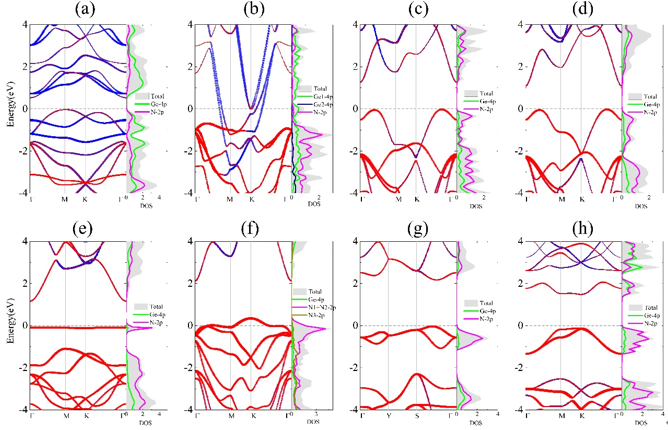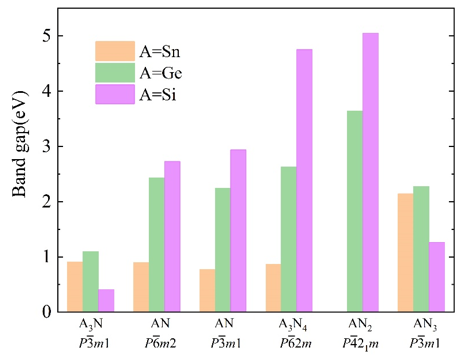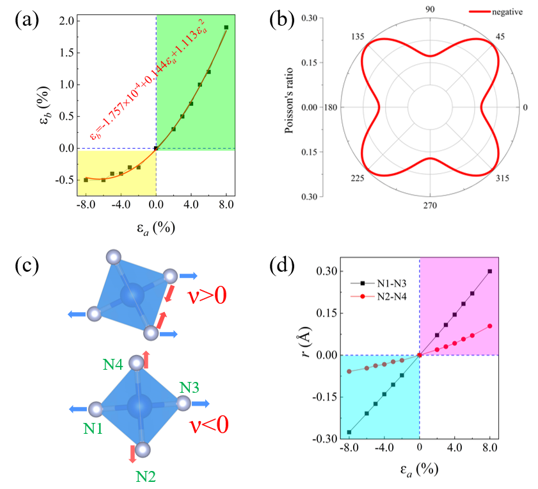Figure 1. Ten 2D prototypes of predicted 2D group IV-nitrides AxNy: (a) C2/m A4N, (b) P3(_)m1 A3N, (c) P3m1 A2N, (d) P3(_)m1 A3N2, (e) P6(_)m2 AN, (f) P3(_)m1 AN, (g) P6(_)2m A3N4, (h) P3m1 A2N3, (i) P4(_)21m AN2, and (j) P3(_)m1 AN3. In (a-j), blue and silver balls indicate A (Sn/Ge/Si) and N atoms, respectively. In (g), A3N2 trigonal bipyramids are indicated using shadow blue triangles.Figure 2. Projected electronic band structures and density of states (DOS) (GGA-PBE level of theory) of 2D GexNy: (a) P3(_)m1 Ge3N, (b) P3(_)m1 Ge3N2, (c) P6(_)m2 GeN, (d) P3(_)m1 GeN, (e) P6(_)2m Ge3N4, (f) P3m1 Ge2N3, (g) P4(_)21m GeN2, and (h) P3(_)m1 GeN3.  In the projected band structures, blue and red colors indicate the energy states contributed by Ge and N atoms, respectively. In (a-h), the Fermi level is set as zero.Figure 3 Calculated band gaps of six semiconducting 2D AxNy protypes at the HSE06 level of theory.

Figure 4. Ferromagnetic properties of 2D P3m1 A2N3: (a) spin charge distribution of the ferromagnetic state; spin-polarized band structures and DOS of (b) 2D P3m1 Sn2N3, (c) 2D P3m1 Ge2N3, and (d) 2D P3m1 Si2N3 at HSE06 level.Figure 5. Negative Poisson’s ratio of 2D P4(_)21m SiN2: (a) The strain response along b direction (εb) versus the strain along a direction (εa); (b) In-plane Poisson’s ratio as a function of the angle θ; (c) Deformation of SiN4 tetrahedra under the external strain; (d) The distance variation (r) of N1-N3, N2-N4 pairs versus εa in the SiN4 tetrahedra. The blue and red arrows in (c) indicate the distance expand and contract, respectively.